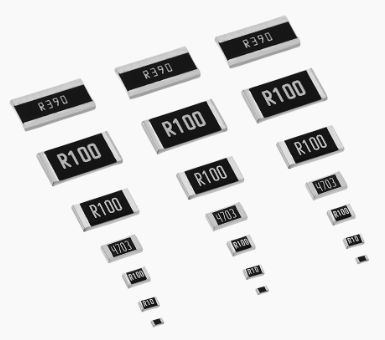How to Read the Value of SMD Resistor? Example Explained
Oct. 18, 2022

SMD Resistor, called Chip Resistor, is one type of resistors. It is a part made by mixing metal powder and glass glaze powder and printing on the substrate by screen printing. It is resistant to humidity and high temperature, and has a small temperature coefficient. Different resistors have different resistance values, for SMD resistors, how are these resistance values marked and read?## What Do Markings on Resistors Mean?

Before acquiring how to read the resistance value, let us first understand how the resistance value is marked, including the four methods:

1. Direct marking method

Use numbers and unit symbols to mark the resistance value on the surface of the resistor, and the allowable error is directly expressed in percentage. If there is no deviation on the resistance, it usually takes ±20%.

2. Text symbol method

Use the regular combination of Arabic numerals and text symbols to indicate the nominal resistance value, and the allowable deviation is also represented by it. The number in front of the symbol indicates the resistance value of an integer, and the number after it indicates the resistance value of the first decimal place and the resistance value of the second decimal place in turn. Character symbols that indicate allowable errors: D-F-G-J-K-M allowable deviations are: ±0.5%, ±1%, ±2%, ±5%, ±10%, ±20%.

3. Digital method

A method of marking the nominal value with three digits on the resistor. The digits are from left to right, the first and second digits are effective values, and the third digit is the exponent, that is, the number of zeros, and the unit is Ω. Deviations are usually expressed in text symbols.

4. Color Code marking method

Mark the nominal resistance value and allowable deviation on the surface of the resistor with bands or dots of different colors, which is common in many regions.

Black-0, Brown-1, Red-2, Orange-3, Yellow-4

Green-5, Blue-6, Violet-7, Gray-8, White-9

Gold-±5%, Silver-±10 %, colorless-±20%

## Resistance Value of the Chip Resistor

The resistance value of the chip resistor is the same as that of a general resistor, which is marked on the resistor body. There are three kinds of resistance nominal methods, but the nominal method is not exactly the same as that of general resistors. The surface of the usual chip resistors is marked with numbers, or represented by letters, and the resistance making method is as follows.

1. The first and second digits represent the significant number of resistance.

2. If the number at the beginning of the third digit is 0, it means tens of ohms (between 10 and 99 ohms). Examples, 100 is 10Ω, and 990 is 99Ω.

3. If the number starting with the third digit is 1, it means several hundred ohms (between 100 and 999 ohms). Examples: 101 is 100Ω, 151 is 150Ω, and 951 is 950Ω.

4. If the number at the beginning of the third digit is 2, it means several thousand ohms (between 1000 and 9999Ω). Examples: 102 is 1K, 152 is 1.5K, and 992 is 9.9K.

5. If the number starting in the third digit is 3, it means tens of K (between 10K and 99K). Examples: 103 is 10K, 223 is 22K, and 993 is 99K.

6. If the number starting in the third digit is 4, it means several hundred K (between 100K and 999K). Examples: 104 is 100K, 204 is 200K, and 854 is 850K.

7. If the number at the beginning of the third digit is 5, it represents a few M (between 1M and 9.9). Examples: 105 is 1M, 155 is 1.5M, and 955 is 9.5M.

8. If the number starting with the third digit is 6, it means ten M (between 100K and 999K). Examples: 106 is 10M, and 566 is 56M.

9. The standard method for four numbers is that the first three digits are real numbers, and the fourth digit is a multiple. Examples: 1001 is 1K, 1002 is 10K, and 1005 is 10M.

In addition, the chip resistors with packages 01005, 0201, and 0402 are too small in size, so there is no marked code, so they can only be measured and distinguished by a multimeter test.